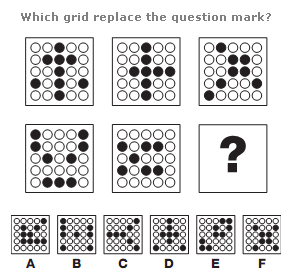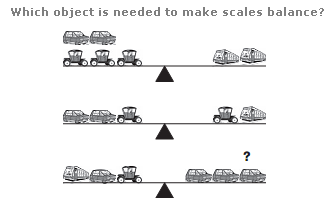# Puzzles - Logical puzzles

### Exercise :: Logical puzzlesAnswer : A Explanation : [NIL]

View Answer & ExplanationAnswer : E Explanation : In each row, the left hand grid is symmetrical around a vertical axis, the central grid is symmetrical about a horizontal axis, and the right hand grid is symmetrical about a diagonal axis, running bottom left to top right.

View Answer & ExplanationAnswer : Carriage Explanation : The Carriage = 2, the Car = 3 and the Bus = 6

View Answer & Explanation

#### Current Affairs 2019

Interview Questions and Answers